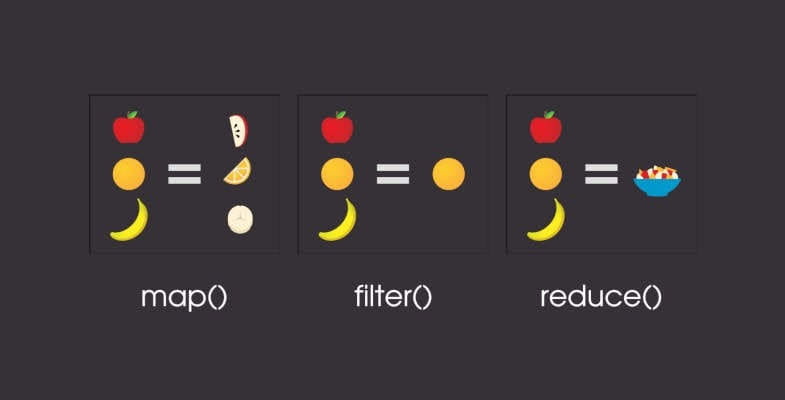# What are the Higher-Order Functions and How to use them in JavaScriptJavaScript is a functional programming that accepts Higher-Order Functions. Higher-Order Functions are widely used in JavaScript.

To understand this concept, you must have to understand what Functional Programming is and the concept of First-Class Functions.

## What is Functional Programming

In simple words, Functional Programming is a form of programming in which you can pass functions as parameters to other functions and also return them as values. In functional programming, we think and code in terms of functions.

JavaScript, Clojure, Haskell, Scala, and Erlang are the languages that implement functional programming.

## First-Class Functions

If you have been learning JavaScript, you may have heard that JavaScript treats functions as first-class citizens. Therefore in JavaScript or any other functional programming languages, functions are objects.

In JavaScript functions are a special type of objects. They are `Function `objects. For example:

``````function welcome() {
console.log('Hello World');
}
// Invoking the function
welcome();  // prints 'Hello World'``````

### Assigning Functions to Variables

We can assign functions to variables in JavaScript. For example:

``````const square = function(x) {
return x * x;
}
// prints 25
square(5); ``````

### Passing Functions as Parameters

We can pass functions as parameters to other functions. For example:

``````function formalGreetings() {
console.log("How are you?");
} function casualGreetings() {
console.log("What's up?");
} function greet(type, greetFormals, greetCasuals) {
if(type === 'formal') {
greetFormals();
} else if(type === 'casual') {
greetCasuals();
}
}
// prints 'What's up?'
greet('casual', formalGreetings, casualGreetings);``````

Now that we know what first-class functions are, let’s jump to Higher-Order functions in JavaScript.

## Higher-Order Functions

Higher-order functions are functions that operate on other functions, either by taking them as arguments or by returning them. In simple words, A Higher-Order function is a function that accepts a function as an argument or returns the function as output.
For example, `Array.prototype.map`, `Array.prototype.filter` and `Array.prototype.reduce` are examples of the Higher-Order functions in JavaScript.

### Array.prototype.map

The `map() `method creates a new array by calling the callback function passed as an argument on every element in the input array. The `map() `method will take every returned value from the callback function and creates a new array using those values.

The callback function passed to the `map() `method accepts 3 arguments: `element`, `index`, and `array`.

Example 1#

Let’s say we have an array of numbers and we want to create a new array that contains double of each value of the first array.

``````const arr1 = [1, 2, 3];
const arr2 = arr1.map(function(element) {
return element * 2;
});
console.log(arr2);``````

We can make this even smaller using the arrow function syntax:

``````const arr1 = [1, 2, 3];
const arr2 = arr1.map(element => element * 2);
console.log(arr2);``````

### Array.prototype.filter

The `filter()` method creates a new array with all elements that pass the test provided by the callback function. The callback function passed to the `filter()` method accepts 3 arguments: `element`, `index`, and `array`.

Example 1#

Let’s say we have an array which contains objects with name and age properties. We want to create an array that contains only the person with full age (age greater than or equal to 18).

``````const persons = [
{ name: 'Kamran', age: 16 },
{ name: 'Hassan', age: 18 },
{ name: 'Asfar', age: 27 },
{ name: 'Kamil', age: 14 },
];
const fullAge = persons.filter(person => person.age >= 18);
console.log(fullAge);``````

### Array.prototype.reduce

The `reduce()` method executes the callback function on each member of the calling array which results in a single output value. The reduce method accepts two parameters: 1) The reducer function (callback), 2) and an optional `initialValue`.

The reducer function (callback) accepts four parameters: `accumulator`, `currentValue`, `currentIndex`, `sourceArray`.

If an `initialValue `is provided, then the `accumulator `will be equal to the `initialValue `and the `currentValue `will be equal to the first element in the array.

If no `initialValue `is provided, then the `accumulator `will be equal to the first element in the array and the `currentValue `will be equal to the second element in the array.

Example 1#

``````const arr = [3, 9, 2, 7, 4];
const sum = arr.reduce(function(accumulator, currentValue) {
return accumulator + currentValue;
});
// prints 25
console.log(sum);``````

Every time the reducer function is called on each value in the array, the `accumulator `keeps the result of previous operation returned from the reducer function, and the `currentValue `is set to the current value of the array. In the end the result is stored in the `sum `variable.

## Conclusion

We have learned what Higher-order functions are and some built-in Higher-order functions. We also learned how to create our own Higher-order functions.